Garrett Mayock's Blog

# Holt-Winters' α,β,γ Model for Seasonal Time Series

### Following along Managing Supply Chain Operations Chapter 2 using Python, cont'd.Garrett Mayock posted 2019-03-16 02:12:13 UTC

As before: I am reading Managing Supply Chain Operations. Chapter 2 details Forecasting and Demand Management methods. I figured I'd kill two birds with one stone (learning the material and learning Python) by recreating the chapter material in Python. I anticipate answering the chapter exercises in Python as well, but will save that for another blog post. (Writer's note - please look at the other Chapter 2 blogs (naive, non-naive, linear regression, Holt's Model, fixing inefficient optimization code, seasonal linear regression models). Click here to download the Excel file I ingested.

Brief note - wow I have been busy! I turned on the LinkedIn "bat signal" - a switch that signals to recruiters that you're open to opportunities - a week ago and I have been swamped. Very encouraging! But a reason I haven't been able to complete a blog post for a week and a half. But I had some time today to finally finish this post up, which finishes the instruction part of Chapter 2. I'll give details on next steps at the bottom.

What is Holt-Winters' model?

Holt-Winters' model is an extension of Holt's model which includes γ as an adjustment factor for updating seasonality. The textbook actually is rather sparse on this topic, with only three sentences in the section for Holt-Winters' model (which they term Winter's model). Furthermore, one of the formulas they include actually has a typo (it refers to St when it means to refer to Gt). It doesn't give any examples, either. Since the textbook has nothing with which I can follow along, I had to make up an example. The first step I did was to recreate one of the data tables in the textbook in the section about adjusting linear regression models for seasonality, which you can see in the 'holt_winters' tab of the Excel file I linked above, or here: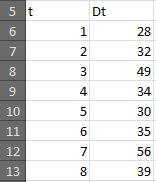Unfortunately, due to the sparse nature of the data (and populating the initial base and growth rate by using linear regression), the model wasn't that much better than linear regression. Optimized [α, β, γ] were [0, 0, 0.012996035894369103], meaning only the γ factor was used (γ is the adjustment factor for new information on the seasonality).

I calculated Mean Squared Error (MSE) to compare the models. Since the way I created the Holt-Winters model forecasts demand for the period ahead, the first period for the Holt-Winters model doesn't have a forecast, so we're only comparing the MSE for periods 2-8. MSE came out with a difference under 1%, which according to my statistical tests is not significant (see statistical test section below).

However, I also checked if I could get a significant difference by optimizing two more variables, and I also applied these models to other data to really show their power.

Replicating the model in Python

Don't get me wrong - the first attempt was technically a "success". The Holt-Winters' model performed ever so slightly better than linear regression. Let's start with the code:

```import pandas as pd

# This ingests just the period and demand data, for the 13 periods

# This renames the columns using a dict
df = df.rename(columns={'t':'Period','Dt':'Demand'})
```

So, it starts off with a similar data import as the regular Holt's model, but then I create lists which I'll use for seasonality:

```# These rows calculate various factors used to calculate seasonality
period_count = len(df['Period'])
cycle_count = 2 # At least one of (cycle_count, season_count_per_cycle) has to be known
season_count_per_cycle = int(round((period_count/cycle_count)+0.5,0))

# This creates lists for raw and average seasonality, of the appropriate legnth
raw_seasonality=[None for i in range(0, period_count)]
avg_seasonality=[None for i in range(0, season_count_per_cycle)]
```

Now, in theory, I could have just input raw data instead of those (period_count, cycle_count, season_count_per_cycle) variables, and saved a few lines. But doing it this way means it's more extensible and means the code that refers to those variables is easier to understand. We'll be seeing a lot of that in the rest of the code, so I think the extra lines are worth it.

Also, in theory, that note I put in which says "At least one of (cycle_count, season_count_per_cycle) has to be known" is probably false, but finding that using code is beyond the current problem scope.

The next step was to calculate seasonality. Note that the textbook teaches us to calculate seasonality using the unadjusted forecast - it would be possible to use the raw demand for that season divided by the sum of raw demand in that cycle, but that ignores the growth factor and would slant seasonality towards the last seasons in a cycle. That means that the first step is to calculate a forecast, which I do using statsmodels.regression.linear_model.OLS:

```# This creates a base forecast
import statsmodels.api as sm
results = sm.OLS(df['Demand'], X).fit()
df['OLS_forecast'] = pd.DataFrame(results.predict(X))
```

Next, I populate those raw and average seasonality lists I previously created with the actual information:

```# This calculates the raw seasonality for each period and replaces the None in the list with the proper value
for i in range(0, period_count):
raw_seasonality[i] = df['Demand'][i]/df['OLS_forecast'][i]

# This averages the raw seasonality across all cycles to get the average seasonality and replaces the None in the list with the proper value
for i in range(0, season_count_per_cycle):
seasonality_factor = 0
for q in range(0,cycle_count):
seasonality_factor = raw_seasonality[i+(q*season_count_per_cycle)] + seasonality_factor
if q == cycle_count-1:
avg_seasonality[i] = seasonality_factor/(q+1)
```

I also want to populate the average seasonality list in the dataframe by making it repeat itself for the length of the dataframe, and then seasonally adjust the OLS forecast and include that as a column as well:

```df['base_seas_fac'] = None
df['SA_OLS_forecast'] = None
# This adds in a Seas Fac and adjusts said Seas Fac for each season
for i in range (0, period_count):
season = i
if season < season_count_per_cycle:
df['base_seas_fac'][season] = avg_seasonality[season]
else:
while season >= season_count_per_cycle:
season = season - season_count_per_cycle
df['base_seas_fac'][i] = avg_seasonality[season]
df['SA_OLS_forecast'] = df['OLS_forecast']*df['base_seas_fac']
```

Note how you can start seeing those variables I previously created being frequency referred to.

The next step is to create the Holt-Winters' model. The first step is to populate the initial seasonality, base, and growth. Since the OLS model calculates a base and growth, I use those as the initial values for the Holt-Winters' model:

```# So now we have the seasonality factors which will can use for our predictions
# Let's set the initial base and growth we'll take from the OLS model
holt_winters_base = results.params
holt_winters_growth = results.params
```

And then define the new Holt-Winters' model, and saying the initial four seasonalities will be the same as the average seasonalities we calculated previously:

```# Now let's define the model
def holtWintersModel(period, alpha, beta, gamma):
global holt_winters_persisted_list
global avg_seasonality
holt_winters_persisted_list = [['Period','HW Base','HW growth','HW unadj Ft for t+1', 'HW Seas Fac','HW Ft for t+1'],[1,holt_winters_base,holt_winters_growth, holt_winters_base+holt_winters_growth*2,avg_seasonality,(holt_winters_base+holt_winters_growth*2)*avg_seasonality]]
for i in range(1, period+1):
if i == 1:
holt_winters_persisted_list[i]
else:
current_demand = df['Demand'][i-1]
last_base = holt_winters_persisted_list[i-1]
last_growth = holt_winters_persisted_list[i-1]
last_forecast = holt_winters_persisted_list[i-1]
if i-1 < season_count_per_cycle:
new_seasonal_factor = df['base_seas_fac'][i]
last_seasonal_factor = df['base_seas_fac'][i-1]
else:
new_seasonal_factor = gamma*(current_demand/new_base) + (1-gamma)*df['base_seas_fac'][i-season_count_per_cycle]
avg_seasonality.append(new_seasonal_factor)
last_seasonal_factor = df['base_seas_fac'][i-1-season_count_per_cycle]
new_base = alpha*(current_demand/last_seasonal_factor)+((1-alpha)*(last_base+last_growth))
new_growth = beta*(new_base-last_base)+(1-beta)*last_growth
holt_winters_persisted_list.append([i,new_base,new_growth,new_base+new_growth*2,new_seasonal_factor,(new_base+new_growth*2)*new_seasonal_factor])
return holt_winters_persisted_list
```

And of course, we need to update the MSE function as well:

```# We can use a very similar MSE function
mse_max = len(df['Period'])
def holtWintersMSE(x):
global holt_winters_persisted_list
sse_val = 0
sse_count = 0
holtWintersModel(mse_max, x, x, x)
for i in range(0,mse_max-1):
global holt_winters_persisted_list
holt_winters_forecast = holt_winters_persisted_list[i+1]
current_demand = df['Demand'][i+1]
sse_val = (holt_winters_forecast-current_demand)**2 + sse_val
sse_count += 1
mse_val = sse_val/sse_count
return mse_val
```

Optimizing the model parameters

From there, it's just setting an initial guess for optimization and printing the results:

```# Let's set the initial α,β,γ values
test_alpha = 0.5
test_beta = 0.5
test_gamma = 0.5
initial_guess = [test_alpha, test_beta, test_gamma]

# And now we optimize the MSE with scipy.optimize.minimize
from scipy.optimize import minimize
result = minimize(holtWintersMSE, initial_guess, bounds=((0,1),(0,1),(0,1)))

# This calculates the MSE for the seasonally adjusted OLS forecast
SA_OLS_MSE = pd.DataFrame([(df['SA_OLS_forecast'][i]-df['Demand'][i])**2 for i in range(1,len(df['Demand']))]).mean()

# This prints results
print("\nOptimized alpha:\n",result.x,"\nOptimized beta:\n",result.x,"\nOptimized gamma:\n",result.x,"\nSA OLS Mean Squared Error\n", SA_OLS_MSE, "\nHolt Winters' Mean Squared Error\n", holtWintersMSE(result.x), "\nMSE difference:\n", holtWintersMSE(result.x)-SA_OLS_MSE, "\nDifference as a ratio:\n", (holtWintersMSE(result.x)-SA_OLS_MSE)/SA_OLS_MSE)
```

If we look at the results, we see the MSE for the linear regression model is only ~0.02 higher than the MSE for the Holt-Winters' model: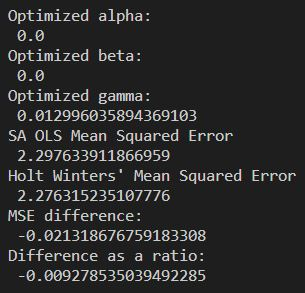We can even add some code to print the dataframe and see the results side by side:

```# This creates a dataframe with all of the information
holt_winters_df = pd.DataFrame(holt_winters_persisted_list[1:], columns=holt_winters_persisted_list)
holt_winters_df['HW Ft'] = holt_winters_df['HW Ft']
new_df = pd.merge(df, holt_winters_df, how='outer', on=['Period'])

# And this prints it
print("\n",new_df)
```

Which looks like: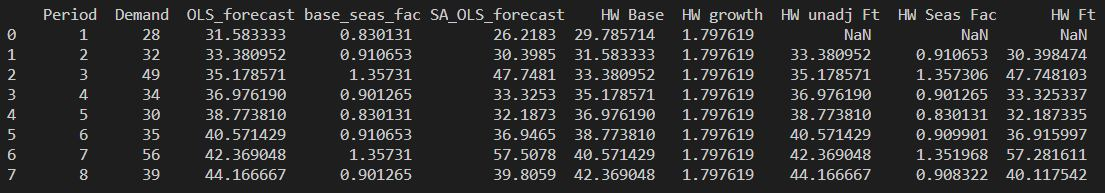Testing statistical significance

I added a quick independent t-test to determine statistical significance of the difference in MSEs, using scipy.stats.ttest_ind():

```# This is a statistical test. If we observe a large p-value, for example larger than 0.05 or 0.1, then we cannot reject the null hypothesis of identical average scores.
from scipy import stats
ttest_results = stats.ttest_ind((new_df['HW Ft'][1:]-new_df['Demand'][1:])**2, (new_df['SA_OLS_forecast'][1:]-new_df['Demand'][1:])**2)
print("\nT-test results:\n",ttest_results)
```

The results indicate that there is nearly a 98% chance that the null hypothesis (which states that there is no significant difference, a.k.a. that any observed difference being due to sampling or experimental error) is true. Usually we don't reject the null hypothesis unless the chance that it's true is under 5% or 10%. This means that the difference in projections between this models on this data set is not statistically significant.

So what gives? Well, I think the data is just too sparse for this model to have a marked improvement over a linear regression model.

Seeing the difference if two more inputs are allowed to be optimized (S0 and G0)

Let's see how it does in another case - in which I change the base and growth to be part of the factors optimized by the SciPy optimization function. To do that, I have to add in the Holt-Winters' base and the Holt-Winters' growth as inputs to the function defining Holt-Winters' model. They will affect only the first year, which is reflected in holt_winters_persisted_list:

```# Now let's define the model *with HW_base and HW_growth as inputs)
def holtWintersModel(period, HW_base, HW_growth, alpha, beta, gamma):
global holt_winters_persisted_list
global avg_seasonality
holt_winters_persisted_list = [['Period','HW Base','HW growth','HW unadj Ft for t+1', 'HW Seas Fac','HW Ft for t+1'],[1,HW_base,HW_growth, HW_base+HW_growth*2,avg_seasonality,(HW_base+HW_growth*2)*avg_seasonality]]
```

And the rest of the code from there on remains the same, so I'll not unnecessarily lengthen the blog by copy/pasting those 19 lines. However, if we add them as inputs to the function defining Holt-Winters' model, we have to add them as inputs into the function which calculates MSE:

```# Only the call to holtWintersModel() changes when adding HW_base and HW_growth, as compared to before
mse_max = len(df['Period'])
def holtWintersMSE(x):
global holt_winters_persisted_list
sse_val = 0
sse_count = 0
holtWintersModel(mse_max, x, x, x, x, x)
```

Again, the code thereafter in the holtWintersMSE() function remains the same, so I've omitted those 8 lines from the blog. The next change then is the initial_guess variable, which we input into the optimize function:

```initial_guess = [holt_winters_base, holt_winters_growth, test_alpha, test_beta, test_gamma]
```

And then we have to add bounds to those variables in the optimize function call:

```# And now we optimize the MSE with scipy.optimize.minimize
from scipy.optimize import minimize
result = minimize(holtWintersMSE, initial_guess, bounds=((0, None), (0, None), (0,1),(0,1),(0,1)))
```

Now, the results here are fairly similar as far as α,β,γ - [0, 0, 0.022186176053203725]. However, the change in initial base and initial growth (to approximately 32.15 and 1.259) resulted in a much lower pvalue: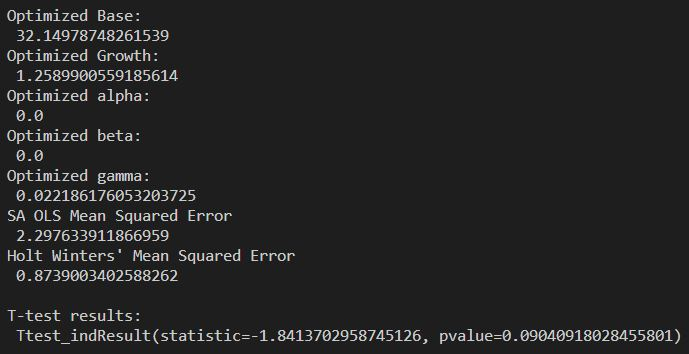This P-value is low enough that we can say with over 90% confidence that the optimizing the base and growth had a significant effect on the results.

Visualizing the differences

Okay, so we know the optimization on three variables (α,β,γ) did not significantly affect the forecast. However, we have 90%+ confidence that the optimization on five variables (initial base, initial growth, α, β, γ) did. Let's see what it looks like! First let's get all the data in a single dataframe:

```# This combines both datasets, drops extra columns, and renames some columns
new_df_3 = pd.merge(new_df, new_df_2, how='outer', on=['Period','Demand','OLS_forecast','base_seas_fac','SA_OLS_forecast'])
new_df_3 = new_df_3.drop(columns=['base_seas_fac','HW Base_x','HW growth_x','HW unadj Ft_x','HW Seas Fac_x','HW Base_y','HW growth_y','HW unadj Ft_y','HW Seas Fac_y'])
new_df_3 = new_df_3.rename(columns={'HW Ft_x':'Three-variable HW Ft','HW Ft_y':'Five-variable HW Ft'})
```

Now let's plot it:

```# Now let's plot the demand and forecasts together
import matplotlib.pyplot as plt
plt.plot(new_df_3['Period'],new_df_3['Demand'], color='blue', linewidth=3.0, label='Actual Demand')
plt.plot(new_df_3['Period'],new_df_3['SA_OLS_forecast'], color='red', linestyle=':', label='Seasonally Adjusted OLS Regression')
plt.plot(new_df_3['Period'],new_df_3['Three-variable HW Ft'], color='orange', linestyle='-', label='Three-variable Holt-Winters\' Forecast')
plt.plot(new_df_3['Period'],new_df_3['Five-variable HW Ft'], color='green', linestyle=':', label='Five-variable Holt-Winters\' Forecast')
plt.xlabel('Period')
plt.ylabel('Demand')
plt.title('Demand by Period (2 cycles)')
plt.legend()
plt.show()
```

And that looks like: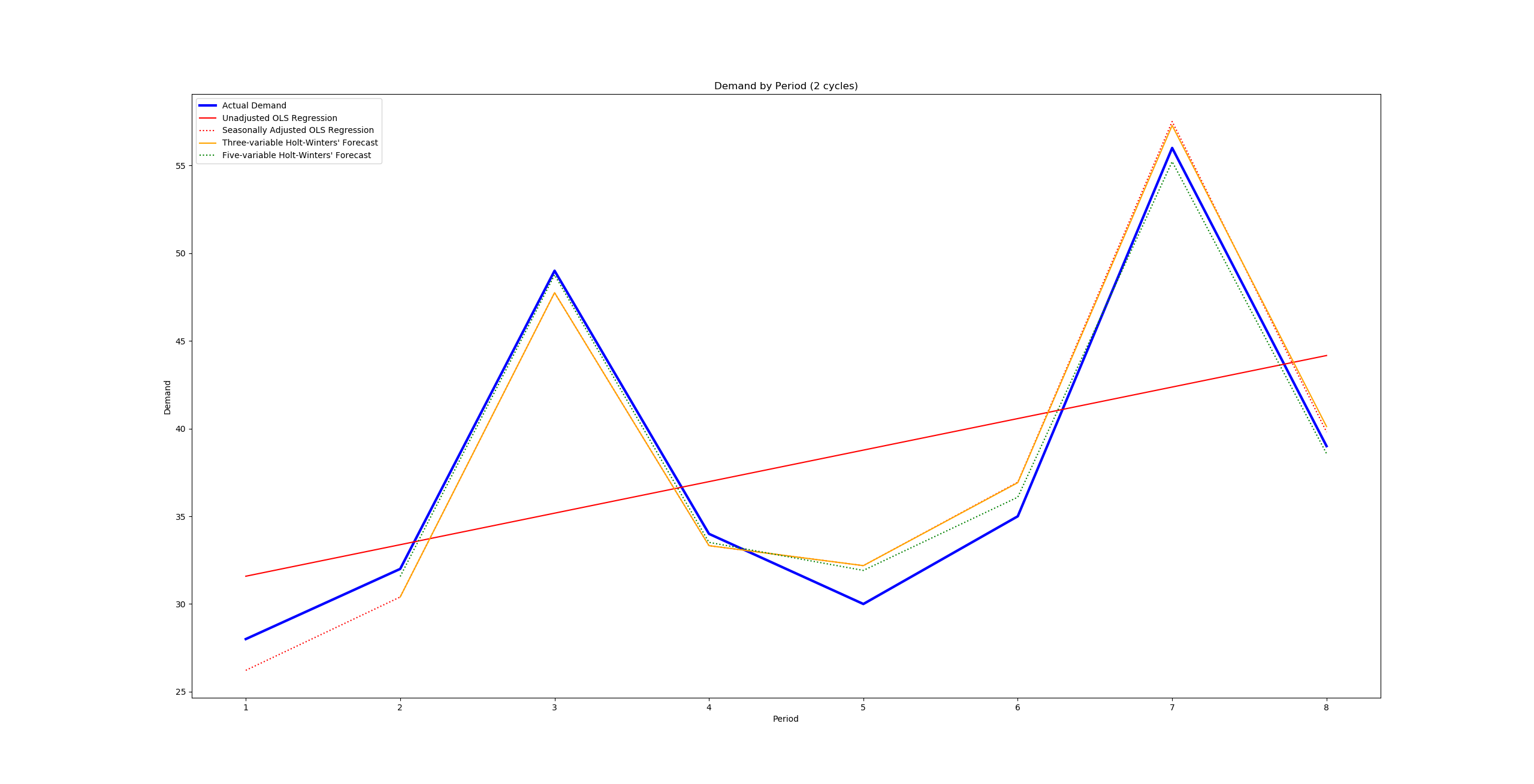Okay, kind of disappointing visualization there, but if we look really closely we can see the five-variable Holt-Winters' forecast stays a lot closer to that blue line representing demand than any of the others. We can also visualize how the three-variable Holt-Winters' forecast doesn't diverge from the seasonally adjusted OLS regression forecast until the second cycle, given it started with the same variables and only changed the seasonality (which doesn't affect it until the next cycle).

Seeing the model's strength with Housing Starts data

Frequent readers may remember the Fed's Housing Starts data I used in the last Python blog. This is where we start to see the real power of this method. I won't be showing the code again, but take a look at a linear regression model over the last 20 years' data: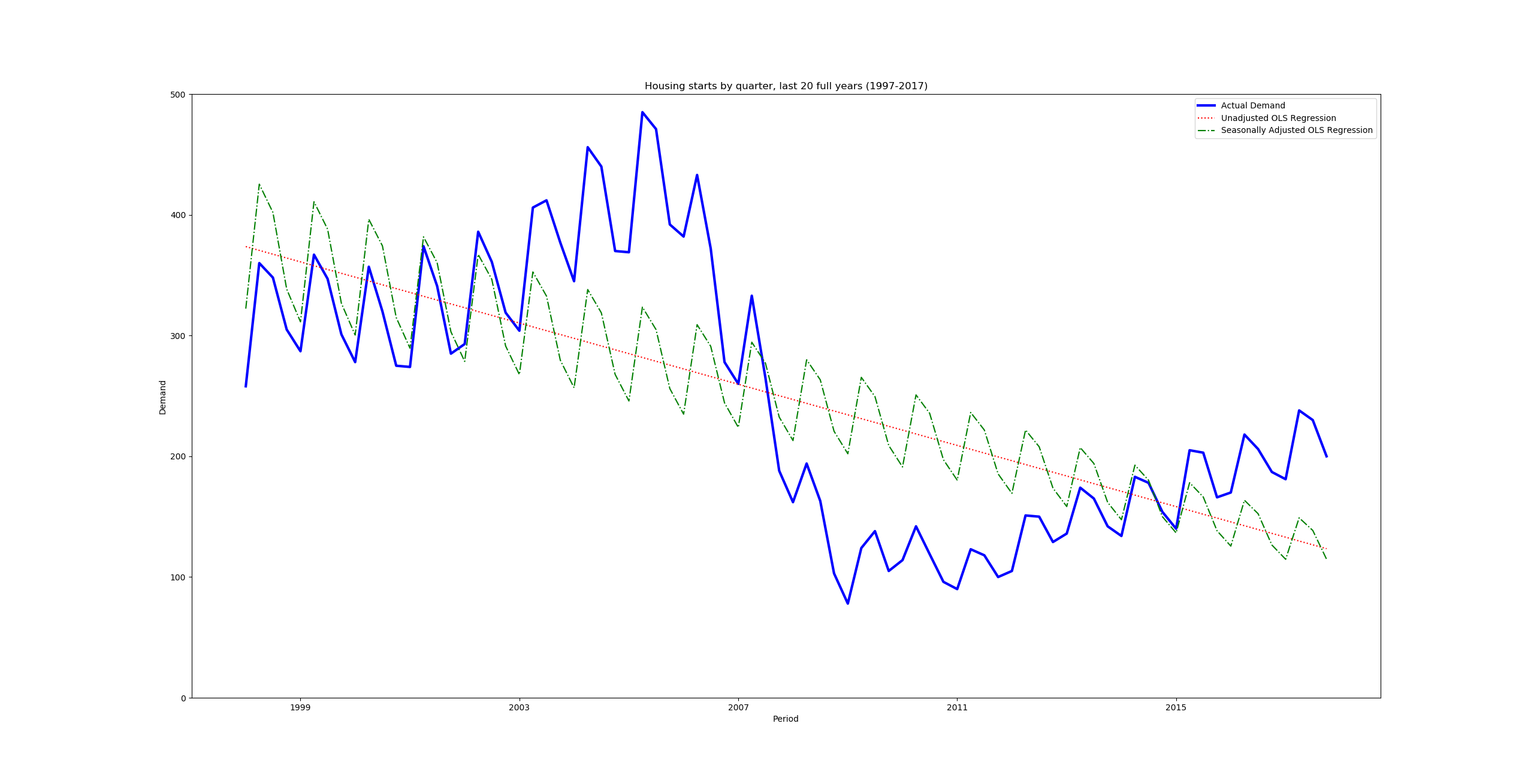And let's see the comparison of the OLS to the three-variable Holt-Winters' model: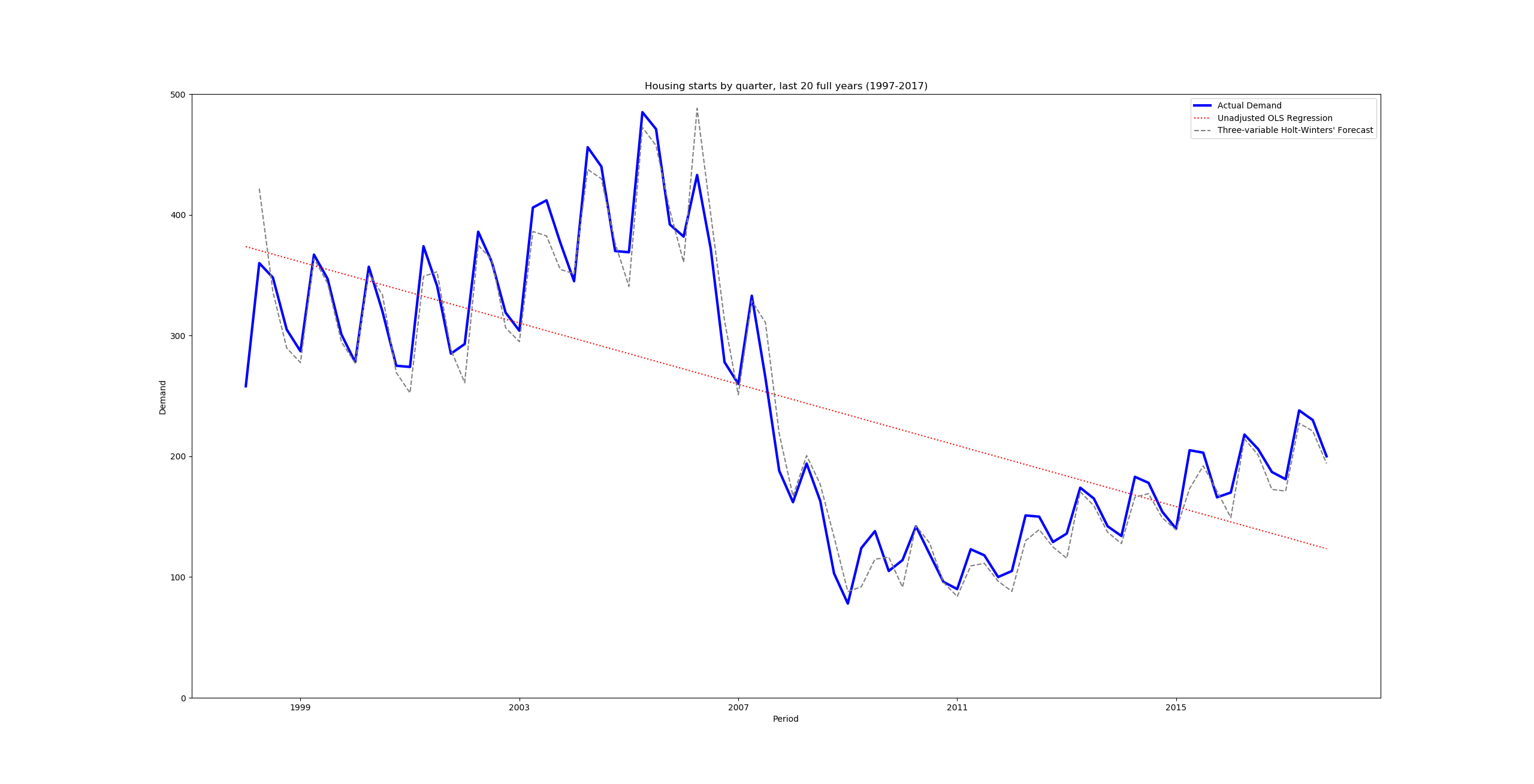And the comparison of the three- and five-variable Holt-Winters' models: